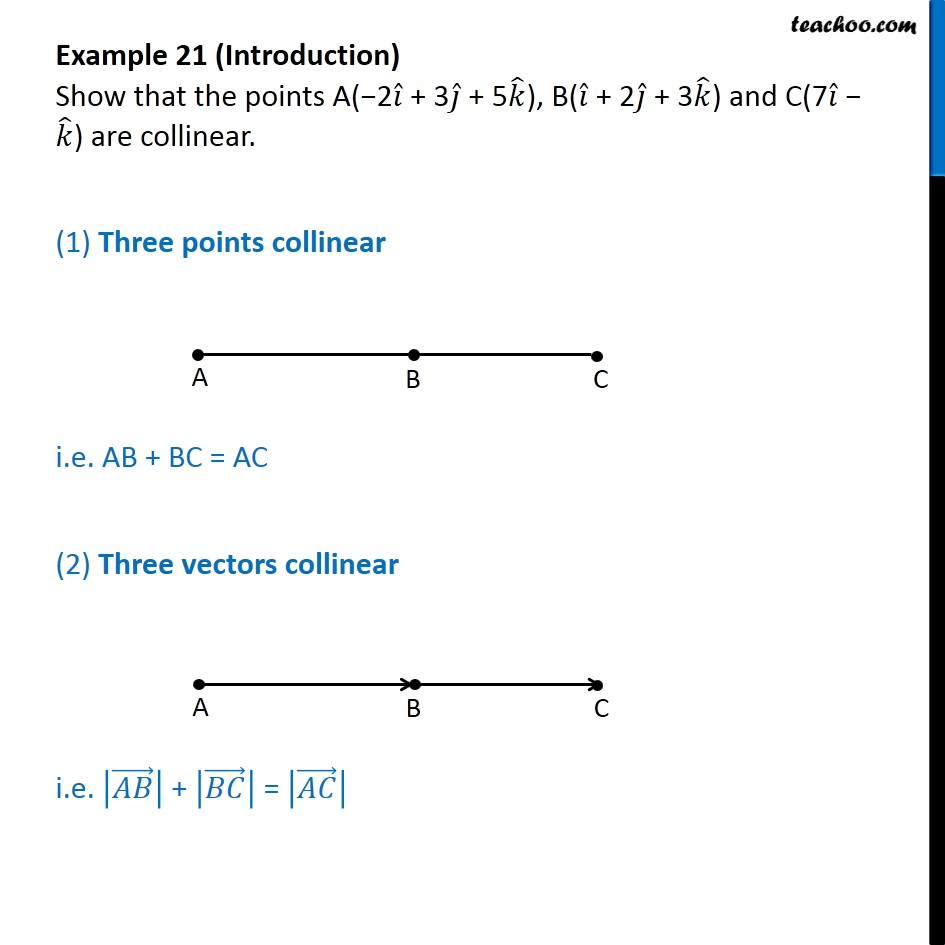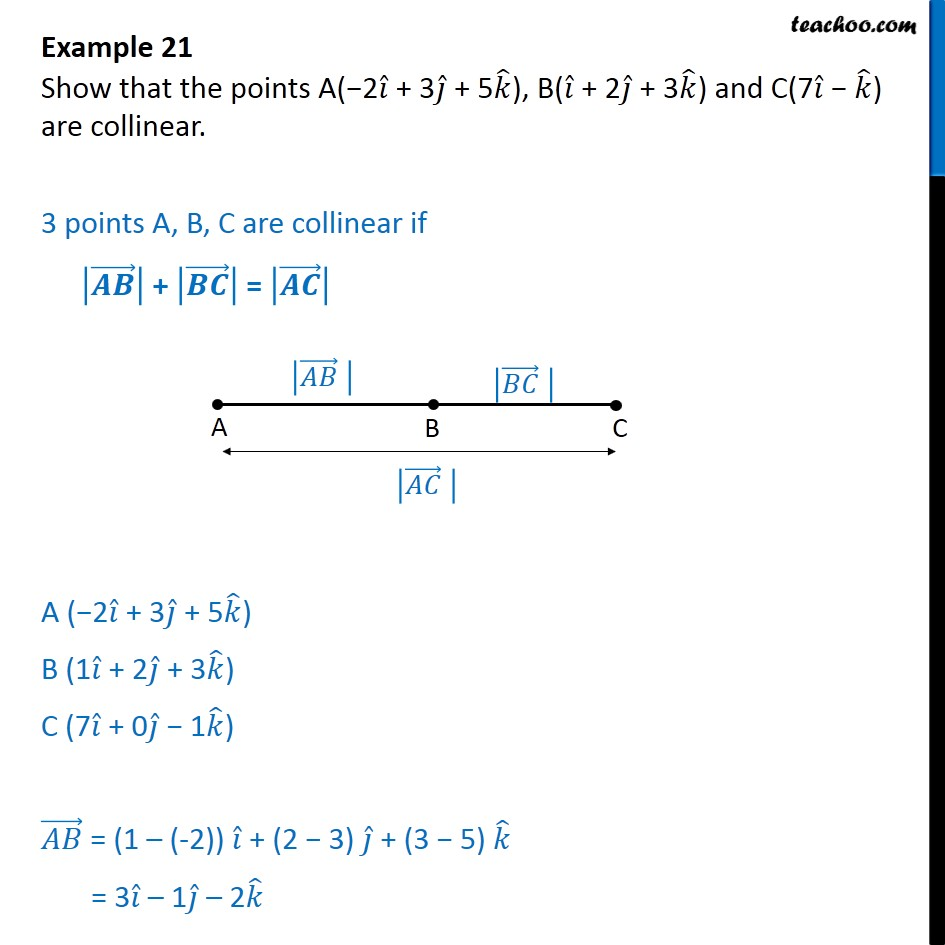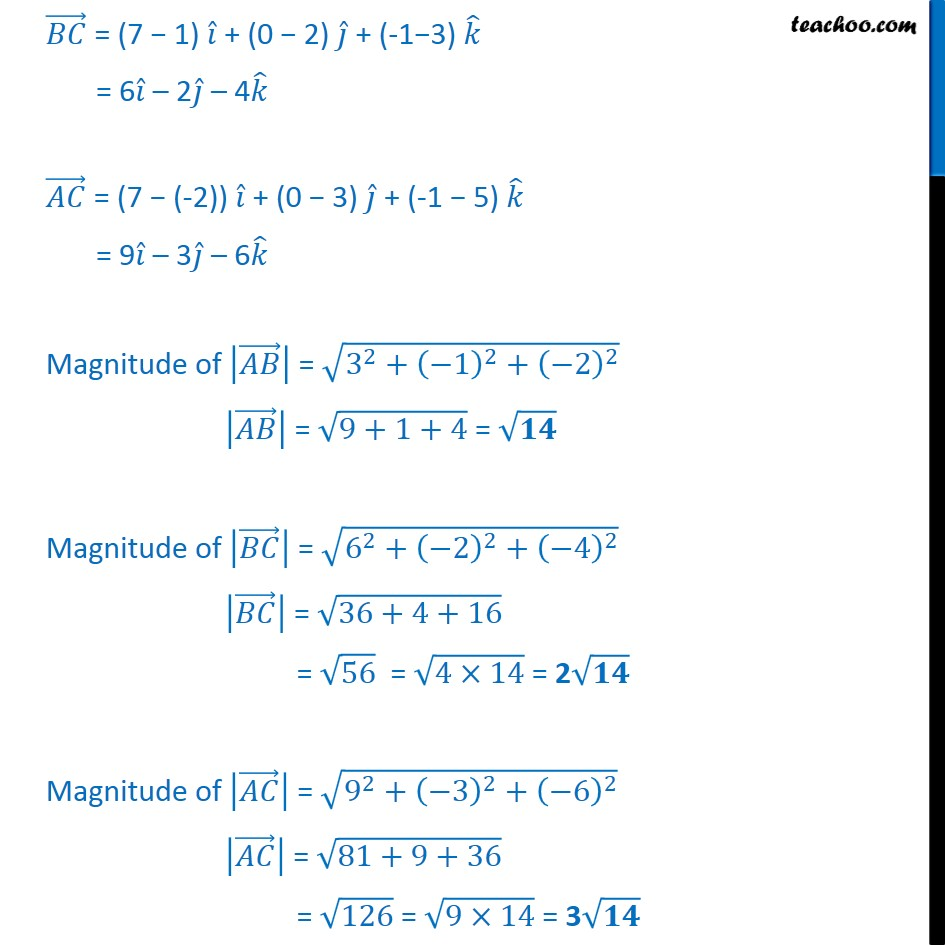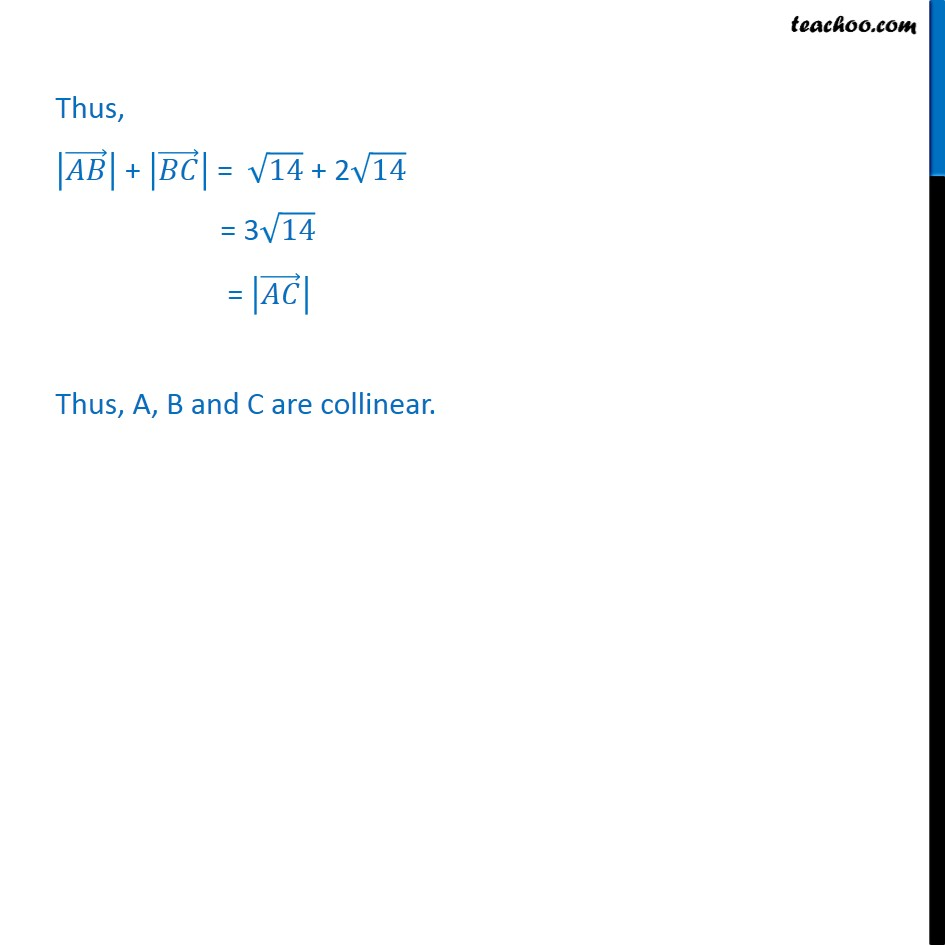1. Class 12
2. Important Question for exams Class 12
3. Chapter 10 Class 12 Vector Algebra

Transcript

Example 21 (Introduction) Show that the points A( 2 + 3 + 5 ), B( + 2 + 3 ) and C(7 ) are collinear. (1) Three points collinear i.e. AB + BC = AC (2) Three vectors collinear i.e. + = Example 21 Show that the points A( 2 + 3 + 5 ), B( + 2 + 3 ) and C(7 ) are collinear. 3 points A, B, C are collinear if + = A ( 2 + 3 + 5 ) B (1 + 2 + 3 ) C (7 + 0 1 ) = (1 (-2)) + (2 3) + (3 5) = 3 1 2 = (7 1) + (0 2) + (-1 3) = 6 2 4 = (7 (-2)) + (0 3) + (-1 5) = 9 3 6 Magnitude of = 3 2 + 1 2 + 2 2 = 9+1+4 = Magnitude of = 6 2 + 2 2 + 4 2 = 36+4+16 = 56 = 4 14 = 2 Magnitude of = 9 2 + 3 2 + 6 2 = 81+9+36 = 126 = 9 14 = 3 Thus, + = 14 + 2 14 = 3 14 = Thus, A, B and C are collinear.

Chapter 10 Class 12 Vector Algebra

Class 12
Important Question for exams Class 12